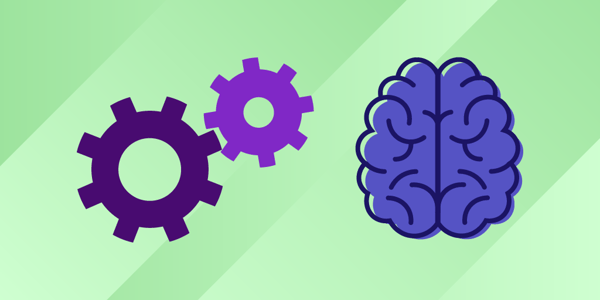# The Complete Beginner’s Guide to Machine Learning with Scikit-Learn# The Complete Beginner’s Guide to Machine Learning with Scikit-Learn

## Introduction

As the field of machine learning continues to evolve, so too does the repertoire of tools that data scientists have at their disposal. Python, a versatile and powerful programming language, is one of the top choices for machine learning, and Scikit-learn is arguably one of its most important libraries. Scikit-learn provides a range of supervised and unsupervised learning algorithms via a consistent interface in Python. This article aims to serve as a comprehensive guide for beginners looking to embark on their machine learning journey with Scikit-learn.

## I. The Scikit-Learn Ecosystem

Before diving into Scikit-learn, it’s important to understand the Python ecosystem it’s part of. Scikit-learn builds upon the core Python scientific stack, which includes:

1. NumPy: The fundamental package for numerical computation in Python, NumPy provides support for arrays, mathematical functions, random number capabilities, and much more.

2. SciPy: A package that builds on NumPy by adding a collection of algorithms and high-level commands for data manipulation and analysis.

3. Matplotlib: A plotting library that provides the capability to create a wide range of static, animated, and interactive plots in Python.

4. Pandas: A library that provides high-performance, easy-to-use data structures, and data analysis tools.

## II. Installing Scikit-Learn

The easiest way to install Scikit-learn is via pip. If you’re using a Jupyter notebook, you can install it in a cell using:

``!pip install -U scikit-learn``

If you’re using a terminal, you can simply drop the exclamation mark:

``pip install -U scikit-learn``

Scikit-Learn includes several popular datasets. For instance, the iris and digits datasets are often used as beginner’s examples for classification tasks. To load a dataset:

``````from sklearn import datasets

``````

## IV. Exploring a Dataset

A Scikit-Learn dataset is a dictionary-like object that holds all the data and some metadata about the data. The dataset’s samples (input data) are stored in the `.data` member, which is a `(n_samples, n_features)` array. In supervised tasks, one or more response variables are stored in the `.target` member.

## V. Building a Model

The process of building a model involves defining the model, fitting it to the data, and then making predictions. For instance, using Scikit-Learn’s support vector classifier looks like this:

``````from sklearn import svm

clf = svm.SVC(gamma=0.001, C=100.)

clf.fit(digits.data[:-1], digits.target[:-1])

clf.predict(digits.data[-1:])
``````

Scikit-Learn models can be persisted (saved) using Python’s built-in persistence model, `pickle`. This can be useful when models take a long time to train, or when you want to save the model for later use:

``````import pickle

s = pickle.dumps(clf)

``````

For larger datasets, it might be more practical to use joblib, a replacement for pickle that’s optimized for large numpy arrays:

``````from joblib import dump, load

dump(clf, 'filename.joblib')

``````

## VII. Scikit-Learn Conventions

Scikit-Learn has established several conventions that help maintain consistency across its API. These include:

Type casting: Unless otherwise specified, input will be cast to `float64`.

Estimators: Any object that can estimate some parameters based on a dataset is called an estimator. The estimation itself is performed by the `fit()` method.

Predictors: Estimators that can generate predictions provide a `predict()` method.

## Conclusion

Scikit-Learn offers an effective way into the world of machine learning in Python. With its diverse suite of machine learning algorithms and consistent API, it enables users to focus on the problem at hand without having to worry about the underlying algorithms’ intricacies. It’s also highly flexible, enabling advanced users to tune and adapt machine learning solutions to their specific needs.

However, as the saying goes, the best way to learn is by doing. To truly master Scikit-Learn, there’s no substitute for rolling up your sleeves and getting your hands dirty with some real-world machine learning tasks.

## Relevant Prompts for Further Exploration:

1. How to install Scikit-Learn on different operating systems?
2. A deeper look into Scikit-Learn datasets.
3. Understanding and implementing different machine learning algorithms in Scikit-Learn.
4. A guide to handling data preprocessing with Scikit-Learn.
5. Using Scikit-Learn for feature extraction and selection.
6. Implementing model selection and evaluation strategies with Scikit-Learn.
7. An in-depth tutorial on Scikit-Learn Pipelines.
8. Solving a real-world problem using Scikit-Learn.
9. How to tune Scikit-Learn models?
10. A guide to handling large datasets with Scikit-Learn.
11. Deploying Scikit-Learn models in production.
12. Using Scikit-Learn for text data.
13. Understanding the mathematics behind Scikit-Learn algorithms.
14. How to contribute to Scikit-Learn’s open-source community?
15. A comparison of Scikit-Learn with other machine learning libraries.

## Find more … …

Machine Learning Project – Machine Learning with scikit-learn in Python

Regression Machine Learning Algorithms in Python with scikit-learn

Cookbook – SWIFT for Beginners – Chapter 38: Style Conventions

Machine Learning for Beginners in Python: Linear Regression Using Scikit-Learn